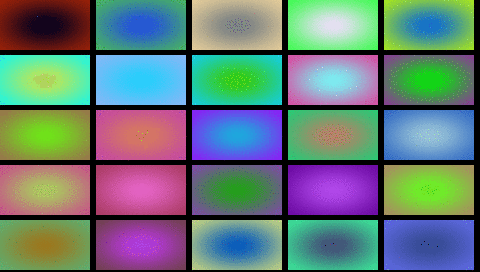The EVE FT81x controllers - used in the Gameduino 3 - can draw linear gradients with a single command. The command specifies two screen points and two colors, and smoothly draws a linear color gradient between them:

```GD.cmd_gradient(0, 0,   0x404044, 480, 480, 0x606068);
```

Circular gradients - also known as radial gradients - are not supported directly. Here are a couple of ways of drawing them.

# Method 1: Pythagorean

For a circular gradient, the color of a pixel is determined by its distance (x, y) from a center:

(x2 + y2)

A bitmap can do the job of computing x2: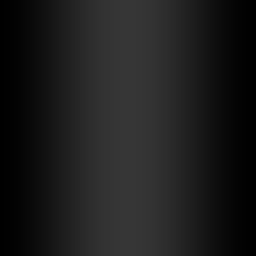By first drawing the x2 then adding the y2 bitmaps, the code renders pixels with brightness proportional to:

x2 + y2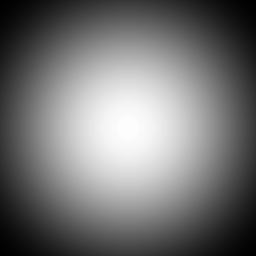Note that the square root term is missing. Because our color perception is ratiometric, this may not be noticeable, depending on the choice of endpoint colors.

After rendering the brightness into the alpha buffer, a further pass applies the gradient colors using destination alpha blending.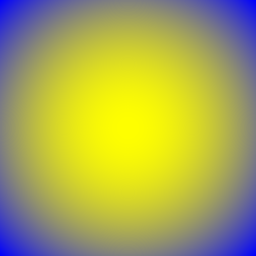# Method 2: Gaussian

Is there a function that can give a circular result from multiplying two linear terms? Only the gaussian function is separable in this way.

So setting the bitmap to a gaussian instead:

f(x) = ae − (x2)/(2c2)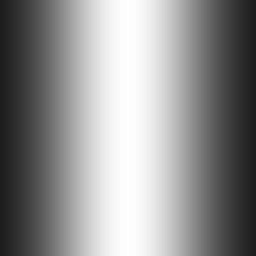and multiplying it with a similar bitmap in y: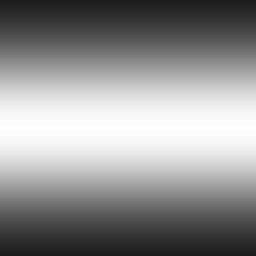gives a two-dimensional gaussian: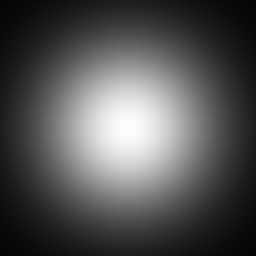and again applying the colors gives a slightly different gradient: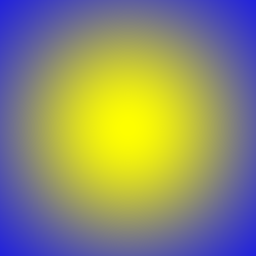For comparison here is the Pythagorean gradient again:The choice is a matter of personal preference. The Pythagorean gradient reaches zero at the edges. The Guassian gradient has a smoother rolloff but does not reach zero at the edge. I have used Gaussians in all the implementation examples.

# Implementation on Gameduino 3

The bitmaps can use a single 512x1 image in L8 (8-bit alpha) format. The two PNG images are pythag.png or gauss.png. The images are converted to GD3 assets:

```gd3asset -o circular_assets.h pythag.png,format=L8 gauss.png,format=L8
```

The main sketch is circular.ino.

Using the function circular you can draw a circular gradient by specifying two corners and two colors:

```circular(0, 0, GD.w, GD.h, 0x772b55, 0xef8619);
GD.cmd_text(GD.w / 2, GD.h / 2, 31, OPT_CENTER, "Hello world");
GD.swap();
```

which drawsThe gradient does not need to fill the screen. For example this code draws randomly colored gradients in 90 x 50 rectangles:

```GD.Clear();
for (int y = 0; y < GD.h; y += 55)
for (int x = 0; x < GD.w; x += 96)
circular(x, y, x + 90, y + 50, rand32(), rand32());
GD.swap();
```

which (slowed down by a factor of 10) gives: